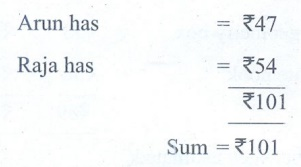Home | | Maths 5th Std | Exercise 2.1 (Estimation)

# Exercise 2.1 (Estimation)

Text Book Back Exercises Questions with Answers, Solution : 5th Maths : Term 3 Unit 2 : Numbers : Exercise 2.1 (Estimation)

Exercise 2.1

1. Fill in the blanks

(i) Estimation of 27 to its nearest Tens place is 30

(ii) Estimation of 65 to its nearest Tens place is 70

(iii) The cost of 1kg pomegranate is ₹ 93. Then the estimated cost is ₹ 90

(iv) Estimation of 76 bananas to its nearest Tens place is 80

2. There are 27 girls and 38 boys in a class. Find the sum and estimate the sum to its nearest Tens place.

Number of girls = 27

Number of boys = 38

27 + 38 =      65

Actual sum = 65

Estimate sum = 70

3. If the cost of a geometry box is ₹ 53 and the cost of a note book is ₹ 36, then estimate their cost to its nearest Tens place and find the sum. Also, find the difference between the estimated value and the actual value.Difference between estimated value and actual value = 90− 89 = ₹1

4. If Kavitha has 93 pictures and she gave 42 pictures to her friend Neela, then estimate the picutres to its nearest Tens place and find their difference. Also, find the difference between actual value and the estimated value.Difference between actual value and estimated value = 51− 50 = 1

5. The cost of a pen is ₹ 32. Find the cost of 6 pens and estimate it to its nearest Tens place.

Cost of a pen = ₹ 32

Cost of 6 pens = 32 ×2 = ₹192

Actual cost = ₹192

Estimated cost = ₹190

6. Arun has ₹47, Raja has ₹ 54. Find the sum and estimate it to its nearest Hundreds place.

Anin has = ₹47

Raja has = ₹54Sum = ₹101

Estimated amount to its nearest hundreds place = ₹100

7. There are 21 chocolate bars in a packet. Find the number of chocolate bars in 9 such packets and estimate it to its nearest Hundreds place.

Number of chocolate bars in a packet = 21

Number of chocolate bars in 9 packets = 21×9

= 189

Actual Number of chocolate = 189

Estimated number of chocolate to its nearest hundreds place = 200

8. 132 peanut candies are shared equally with 12 students. Find the share for one student and estimate it to its nearest Tens place.

Total number of peanut = 132

Total number of students = 12

Share for one student . = 132 ÷ 12

= 11

Actual number of peanut = 11

Estimated number of peanut = 10

Exercise 2.1

1. (i) 30 (ii) 70 (iii) 90 (iv) 80

2. 70

3. 90; 1

4. 50;1

5. 160

6. 100

7. 190

8. 11;10

Tags : Numbers | Term 3 Chapter 2 | 5th Maths , 5th Maths : Term 3 Unit 2 : Numbers
Study Material, Lecturing Notes, Assignment, Reference, Wiki description explanation, brief detail
5th Maths : Term 3 Unit 2 : Numbers : Exercise 2.1 (Estimation) | Numbers | Term 3 Chapter 2 | 5th Maths